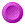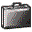` Dmitry Pelinovsky Web Page: Publication Bank

# Stability of nonlinear waves

 Invited papers:D. Pelinovsky and J. Yang, Stability analysis of embedded solitons in the generalized third-order NLS equation, Chaos 15, `037115` (2005)D.E. Pelinovsky and R.H.J. Grimshaw, Asymptotic Methods in Soliton Stability Theory, Advances in Fluid Mechanics Series, 12: Nonlinear Instability Analysis, edited by L.Debnath and S.R.Choudhury, (Computational Mechanics Publications, Southampton, Boston), `245-312` (1997) Regular articles:P. Bizon, D. Hunik-Kostyra, and D.E. Pelinovsky, Ground state of the conformal flow on S3 Communications in Pure and Applied Mathematics 72, `1123-1151` (2019)A. Contreras, D.E. Pelinovsky, and M. Plum, Orbital stability of domain walls in coupled Gross-Pitaevskii systems, SIAM Journal of Mathematical Analysis 50, `810-833` (2018)S. Alama, L. Bronsard, A. Contreras, and D.E. Pelinovsky, Domains walls in the coupled Gross-Pitaevskii equations, Archives for Rational Mechanics and its Applications 215, `579-610` (2015)D.E. Pelinovsky and A. Stefanov, Asymptotic stability of small gap solitons in the nonlinear Dirac equations, Journal of Mathematical Physics 53, `073705 (27 pages)` (2012)M. Chugunova and D. Pelinovsky, Count of eigenvalues in the generalized eigenvalue problem, Journal of Mathematical Physics 51, `052901 (19 pages)` (2010)M. Chugunova and D. Pelinovsky, On quadratic eigenvalue problems arising in stability of discrete vortices, Linear Algebra and its Applications 431, `962-973` (2009)M. Chugunova and D. Pelinovsky, Two-pulse solutions in the fifth-order KdV equation: rigorous theory and numerical approximations, Discrete and Continuous Dynamical Systems, Series B 8, `773-800` (2007)A. Comech, S. Cuccagna, and D. Pelinovsky, Nonlinear instability of a critical traveling wave in the generalized Korteweg - de Vries equation, SIAM Journal on Mathematical Analysis 39, `1-33` (2007)M. Chugunova and D. Pelinovsky, Block-diagonalization of the symmetric first-order coupled-mode system, SIAM Journal of Applied Dynamical Systems 5, `66-83` (2006)V. Vougalter and D. Pelinovsky, Eigenvalues of zero energy in the linearized NLS problem, Journal of Mathematical Physics 47, `062701` (2006)S. Cuccagna, D. Pelinovsky, and V. Vougalter, Spectra of positive and negative energies in the linearized NLS problem, Comm. Pure Appl. Math. 58 `1-29` (2005)S. Cuccagna and D. Pelinovsky, Bifurcations from the end points of the essential spectrum in the linearized NLS problem, J. Math. Phys. 46 `053520` (2005)D.E. Pelinovsky, Inertia law for spectral stability of solitary waves in coupled nonlinear Schrodinger equations, Proc. Roy. Soc. Lond. A 461 `783-812` (2005)D.E. Pelinovsky and J. Yang, Instabilities of multihump vector solitons in coupled nonlinear Schrodinger equations, Stud. Appl. Math. 115 `109-137` (2005)Y. Kodama and D. Pelinovsky, Spectral stability and time evolution of N solitons in KdV hierarchy, J. Phys. A: Math. Gen. 38 `6129-6140` (2005)A.Comech and D. Pelinovsky, Purely Nonlinear Instability of Standing Waves with Minimal Energy, Comm. Pure Appl. Math. 56 `1565-1607` (2003)Y. Tan, J. Yang, and D.E. Pelinovsky, Semi-stability of embedded solitons in the general fifth-order KdV equation, Wave Motion 36, `241-255` (2002)D.E. Pelinovsky and J. Yang, A normal form for nonlinear resonance of embedded solitons, Proc. R. Soc. Lond. A 458, `1469-1497` (2002) Proceedings papers:D.E. Pelinovsky, Spectral stability of nonlinear waves in KdV-type evolution equations, "Nonlinear Physical Systems: Spectral Analysis, Stability, and Bifurcations" (Edited by O.N. Kirillov and D.E. Pelinovsky) (Wiley-ISTE, NJ) `377-400` (2014)T. Mizumachi and D. Pelinovsky, On the asymptotic stability of localized modes in the discrete nonlinear Schrodinger equation, Discrete and Continuous Dynamical Systems Series S 5, `971-987` (2012)